# Some experiments with circle-based art

The header of this site is made of tiling squares in slightly varying shades of red. It’s meant to be subtle, but I think it still gives the site a unique look:I think of this as the “new” header, even though my screenshot library tells me I added it in 2016 (!), as does the blog post I wrote about how I make these images.

It has a lot of straight edges, and for a while I’ve been pondering alternatives. A lot of my current designs have swapped right angles for rounded corners; I’m a heavy user of the border-radius property. Could I do something with circles instead of squares?

I made a few prototypes tonight, and for now the squares are staying – but I thought I’d share my experiments. These graphics don’t work for me, but they might work for somebody else.

I started by refamiliarising myself with Pillow, the Python library I use for doing simple graphics. The API has changed since my post in 2016 – I don’t remember what I was using back then, but today I’m using Pillow 9.2.0.

I started with a single red circle, to get the hang of the new `ImageDraw` API:

``````from PIL import Image, ImageDraw

im = Image.new('RGB', size=(500, 500))
draw = ImageDraw.Draw(im)

draw.ellipse([(100, 100), (200, 200)], fill=(255, 0, 0))

im.save('red_circle.png')
``````

Not especially exciting: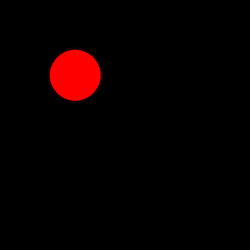I’m passing two arguments to the `ellipse()` method: an xy-bounding box, and a fill colour as an RGB tuple. Because the bounding box is a square, the ellipse becomes a circle – this took me a few moments to figure out, because the word “circle” doesn’t appear anywhere in the ImageDraw docs.

I extended this code to draw circles in a grid, with a random shade of red in each circle:

``````import random
from PIL import Image, ImageDraw

im = Image.new('RGB', size=(500, 500))
draw = ImageDraw.Draw(im)

for x in range(-100, 600, 70):
for y in range(-100, 600, 70):
draw.ellipse(
[(x, y), (x + 100, y + 100)],
fill=(random.randint(128, 255), 0, 0)
)

im.save('grid_of_circles.png')
``````

The circles are drawn left-to-right, top-to-bottom. In one prototype, I accidentally saved an image on every iteration of the xy loop, so you can see how it’s created: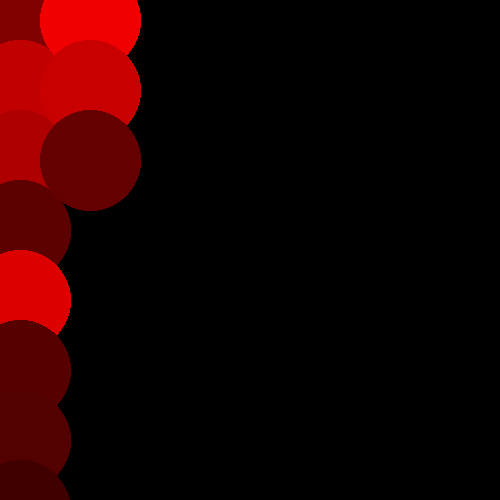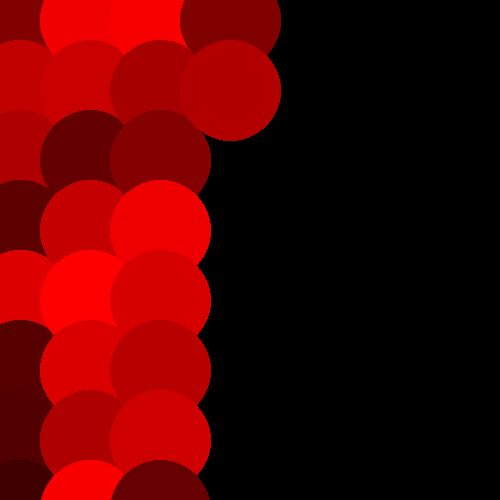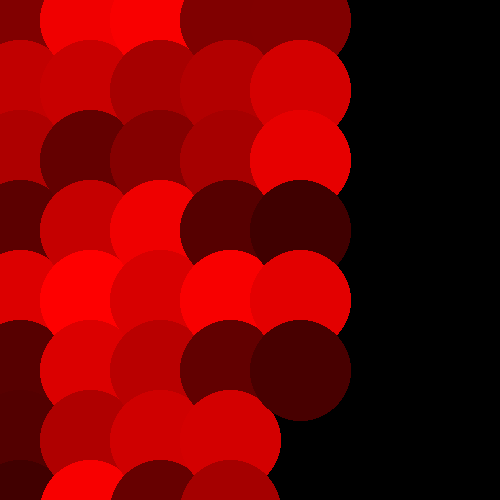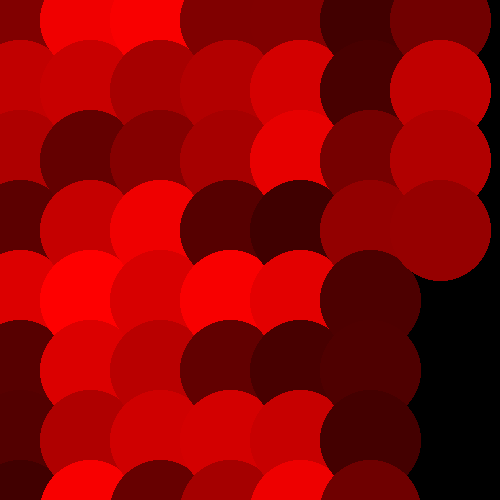Each circle is 100 points in diameter, so I spaced their centres 70 points apart – this is as far as you can space them before some of the background starts poking through in the gaps. (You can work out the exact value with Pythagoras’ theorem, or just tweak the numbers until it looks right.)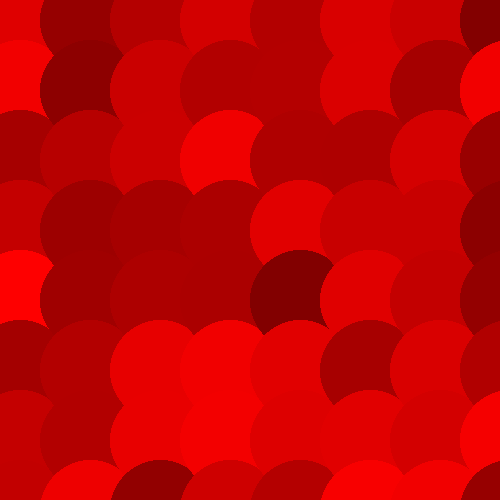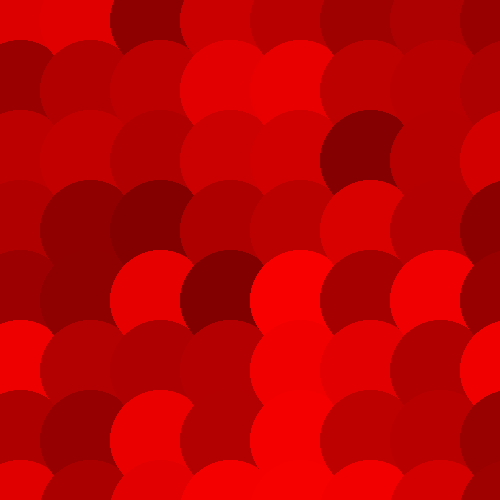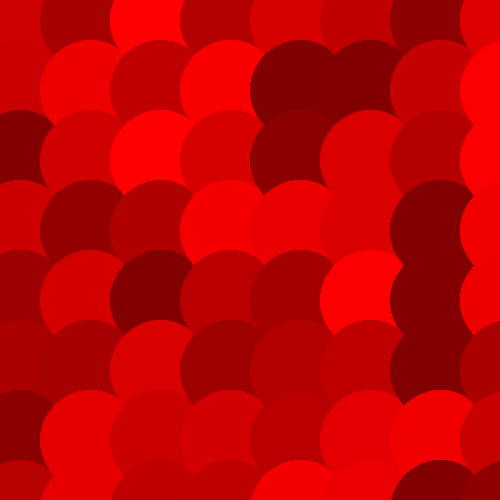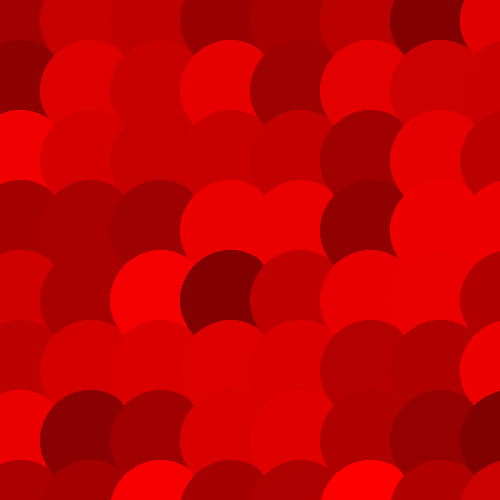The colour and the overlapping shape vaguely remind me of roof tiles – and when I googled, I learnt that this shape is called “fish scale” roof tiles. Yeah, I can see that.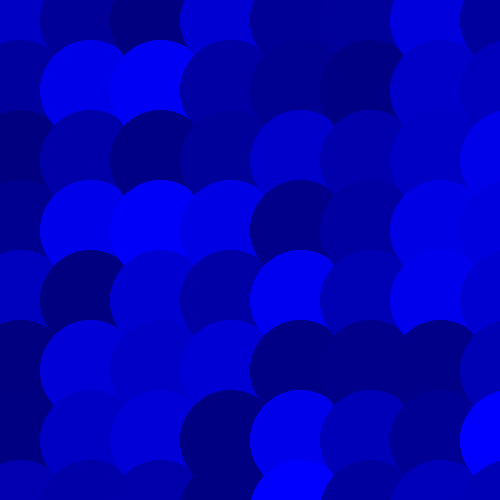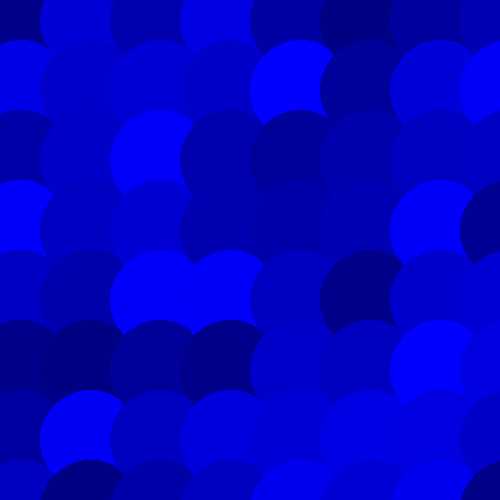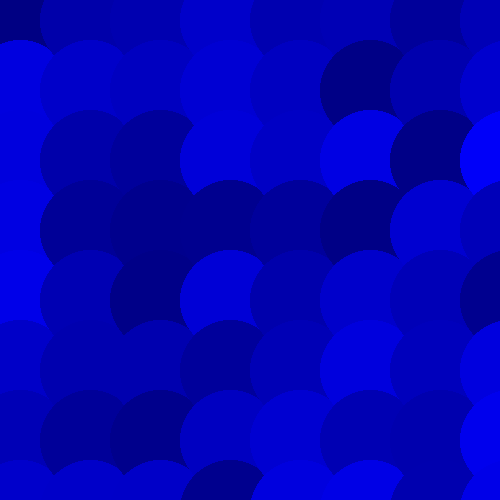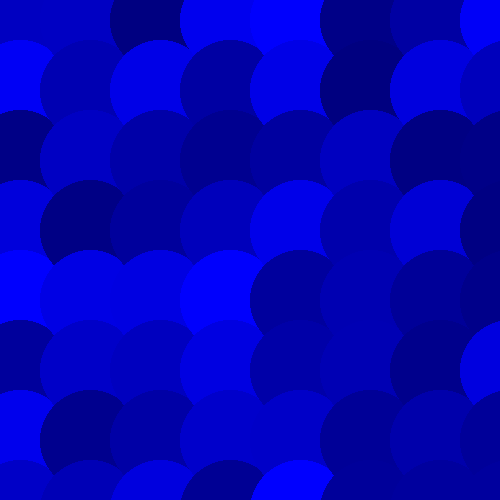But these shapes have a sense of direction – because the circles always overlap on the same two sides, you can see the order in which they were laid down.

I tried shuffling the order in which the circles were drawn:

``````import random
from PIL import Image, ImageDraw

coords = [
(x, y)
for x in range(-100, 600, 70)
for y in range(-100, 600, 70)
]

random.shuffle(coords)

im = Image.new('RGB', size=(500, 500))
draw = ImageDraw.Draw(im)

for (x, y) in coords:
draw.ellipse(
[(x, y), (x + 100, y + 100)],
fill=(random.randint(128, 255), 0, 0)
)

im.save('grid_of_random_circles.png')
``````

These images don’t have a sense of direction, but they’re a bit busy to my eye. Although there’s no pattern, I can feel my brain trying to find one in the various curves: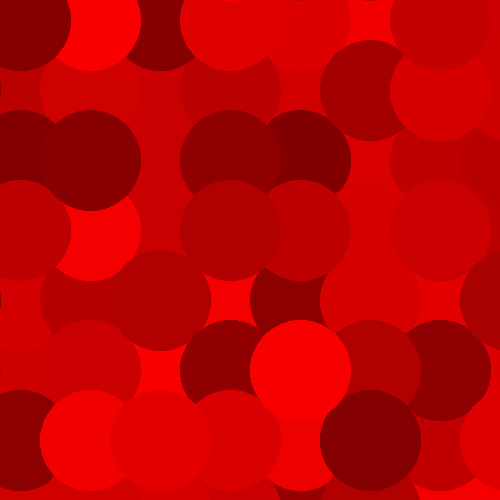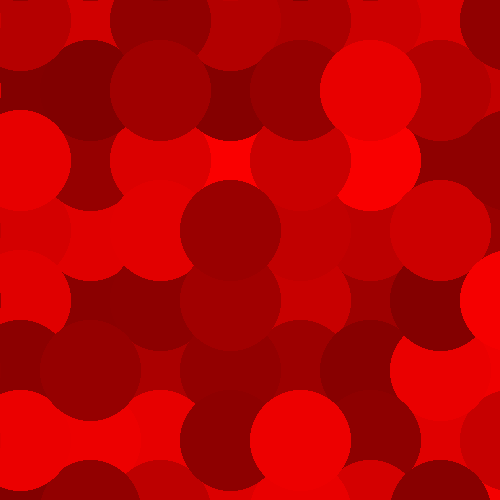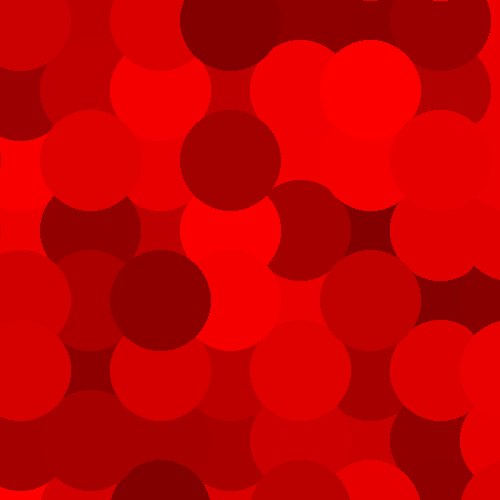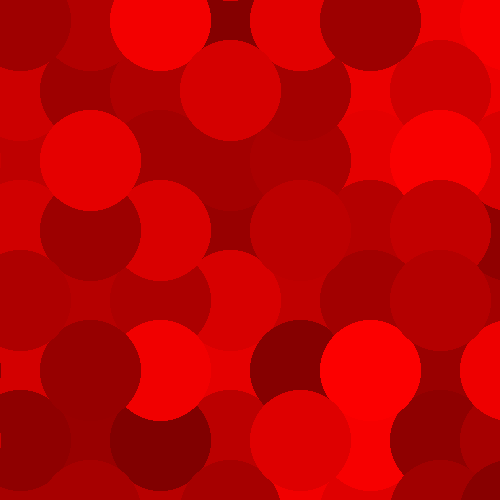I tried one more variation, with more randomness. Rather than drawing equal-sized circles on a fixed grid, what if I drew circles of varying sizes at random points on the grid?

This is what I came up with:

``````import random
from PIL import Image, ImageDraw

im = Image.new('RGB', size=(500, 500))
draw = ImageDraw.Draw(im)

circle_count = 0

while (0, 0, 0) in im.getdata():
x = random.randint(-100, 600)
y = random.randint(-100, 600)
r = random.randint(50, 100)

draw.ellipse(
[(x, y), (x + r, y + r)],
fill=(random.randint(128, 255), 0, 0)
)

circle_count += 1
if circle_count >= 100:
break

print(circle_count)

im.save('random_circles.png')
``````

This draws circles of different radii, centred anywhere on the grid. It will stop after there are no more black pixels (which means circles cover the entire image), or after 100 circles (I wasn’t sure if it this would cover the image in a sensible amount of time). The results are quite nice, and feel vaguely art-like: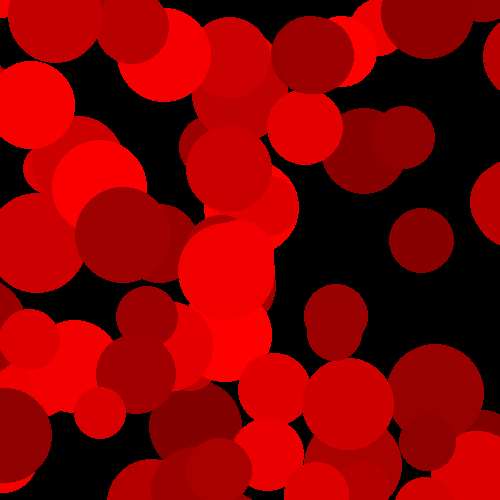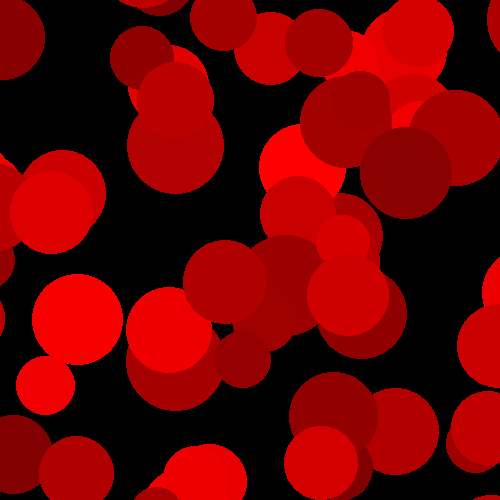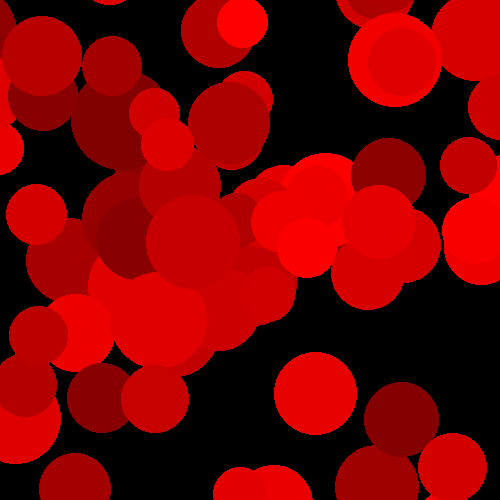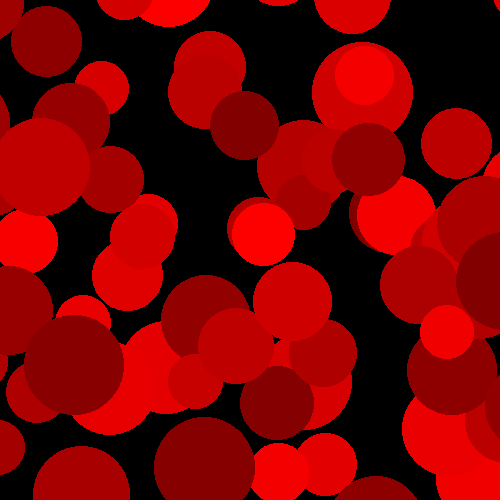I did a batch where I let it pick arbitrary bright colours, and that’s fun too: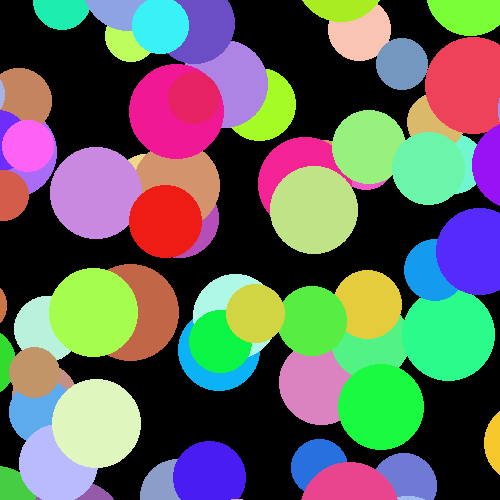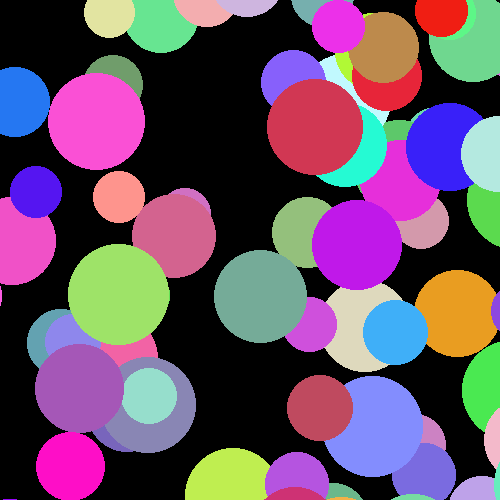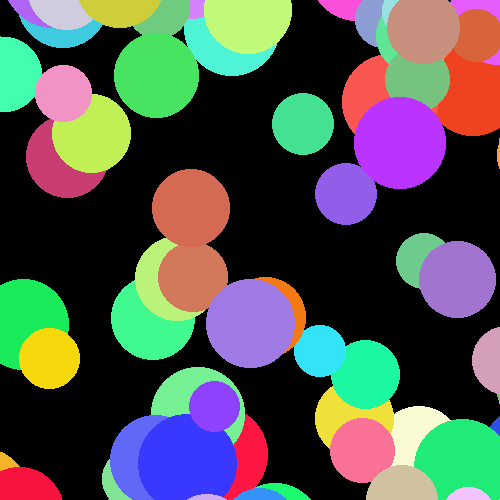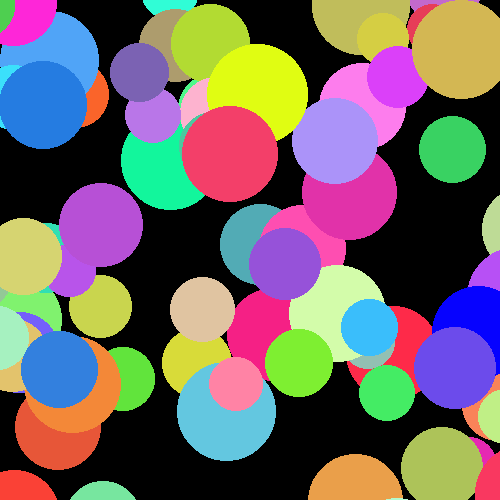I decided to let it run to completion, and keep drawing circles until the whole image was covered. It does eventually complete – the exact number of circles varies, with an average of ~850.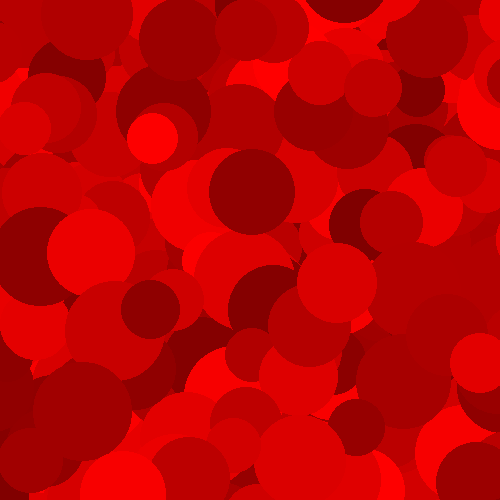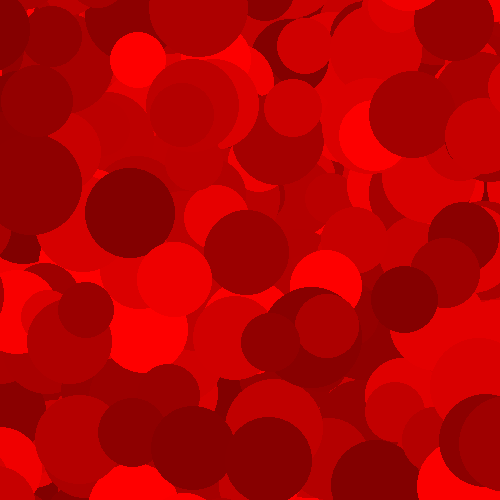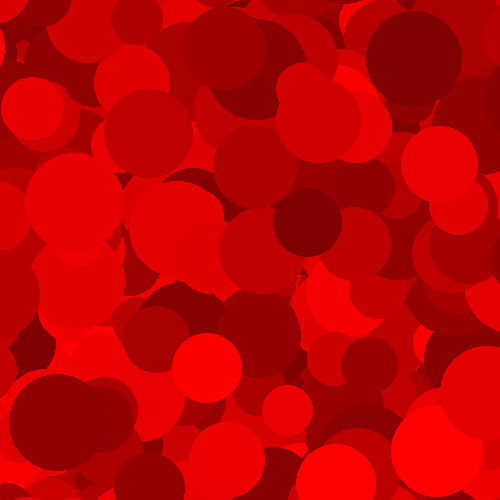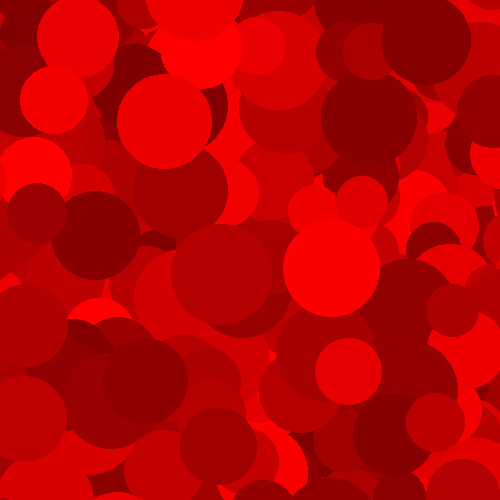This still feels quite busy and loud, and not really what I want in the site header, so I decided to stop here. I still like these images, they’re just not right for this purpose.

One of the things I enjoy about Python and its drawing libraries is how fast I can go from idea to pretty picture. I thought about this on the walk home from the station, and within ten minutes of walking through my door I had the final set of images. It’s a fun creative outlet – and unlike other forms of computer-based art, my randomly generated graphics don’t have any ethical quagmires.